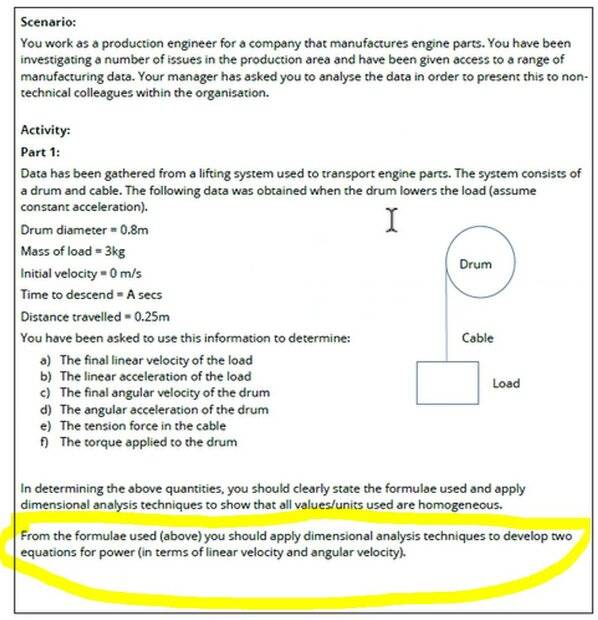# Solve Angular Velocity & Acceleration - Dimensional Analysis

• etile

#### etile

Homework Statement
university
Relevant Equations
n/a
Hi guys,

Please see attached image - it's the part highlighted yellow that I'm stuck on.

Here is what I got for linear velocity and angular velocity. (requested by mod)

Thanks!

c) Angular to velocity
v (linear velocity) = r (radius of circle) * omega (angular velocity)
lets rearrange
linear velo (2.214 m/s = 0.4m* angular)
2.214 / 0.4

d) Angular acceleration of the drum
initial angular velo = 0
final angular veloc=5.535
time=0.225756
formula=
angular acceleration = initial angular velo. (0) - final angular acc. (5.535) / t (0.225756)Last edited by a moderator:
PF rules require you to provide your own efforts. What are your thoughts on the part of the problem you are asking about?

•topsquark
Sorry I didn't realise that.

I pretty much don't understand the question and where to go with it

•